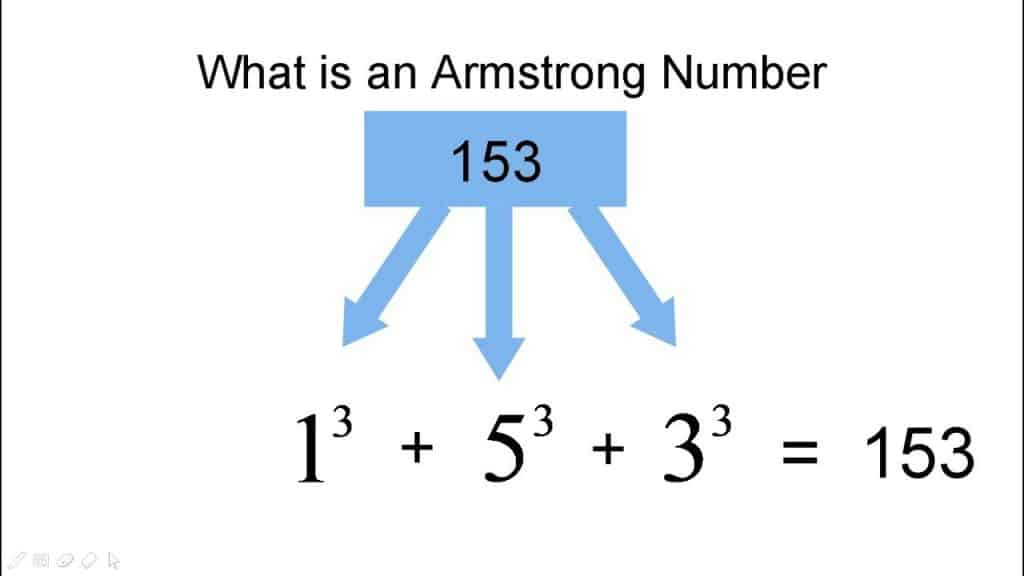# Armstrong Number In Java Programming Language

Armstrong Number In Java is the number that is equal to the sum of all digits to the power of the total number of digits in that number. For example 0, 1, 153, 370, 371, and 407, etc are some of the Armstrong numbers.

In number theory, a narcissistic number, an Armstrong number & it is named after Michael F. Armstrong is a number that is the sum of its own digits each raised to the power of the number of digits.

To understand better check this example given below:Example 1: The Number 153 which is an Armstrong number.

Let’s prove that this number is Armstrong number:

Total digits in number = 3

1^3 = 1*1*1 = 1

5^3 = 5*5*5 = 125

3^3 = 3*3*3 = 9

S0, 153 = (1*1*1)+(5*5*5)+(3*3*3)

153= 1+125+27 , hence proved

Example 2: The Number 370 is an Armstrong number.

Let’s prove that this number is Armstrong number:

Total digits in number = 3

3^3 = 3*3*3 = 27

7^3 = 7*7*7 = 343

0^3 = 0*0*0 = 0

S0, 370 = (3*3*3)+(7*7*7)+(0*0*0)

370= 27+343+0 , hence proved

### Armstrong Number In Java Program Code:

Here we will write a program in java that checks whether the given number is Armstrong number or not.

```public class Armstrong {

public static void main(String[] args) {

int num = 371, originalNum, rem, result = 0;

originalNum = num;

while (originalNum != 0)
{
rem = originalNum % 10;
result += Math.pow(rem, 3);
originalNum /= 10;
}

if(result == num)
System.out.println(num + " is an Armstrong number.");
else
System.out.println(num + " is not an Armstrong number.");
}
}```

Also Check: Armstrong Number In C

Still having difficulty understanding, then you can watch this video to understand the Armstrong number program:

Few easy & quick tricks explained in this video & you can implement them easily as well.

### Conclusion:

You can check the source code of this program in this article itself. Feel free to contact us if you have any questions regarding this program or anything else.